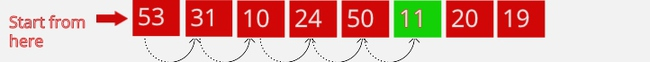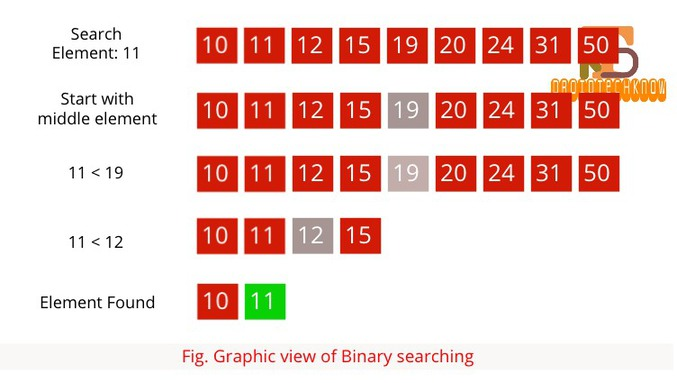# Introduction To Searching Algorithms - Linear Search | Binary Search

April 01, 2020 2815 GulfamIn computer Science, Searching is a technique to find a particular record from the list of records. Just like you always do search for something like keys, mobile, etc in our day to day life. What if you will find everything in place then you will save your time. Similarly, We need to save the computer time by using some efficient searching algorithms which can search a particular record in a short interval of time.

## Searching Techniques

Some of the standard searching algorithms that we used.

• Linear Search or Sequential Search

• Binary Searching

• Interpolation Search

## Linear Search or Sequential Search

Linear search is the most basic searching algorithm to find out a particular element in the list of elements. It traverses the complete collection of data from starting and compare each element of the data with the desired key(Value to be searched). It found the searched element in linear time.In the above figure, Element (11) is compared with each and every element of the array sequentially to check whether 11 exists in the array or not.

### Linear Search Implementation Using JavaScript

function linearSerach(array, item) {
for(var index in array) {
if (array[index] == item) {
return index;
}
}
return1;
}

### Features of Linear search

• Time Complexity: O(N)

• It is very easy to implement.

• It works for ordered as well as an unordered list of elements.

## Binary Searching

The binary searching algorithm is the most efficient and most popular searching algorithm. This algorithm is used to divide and conquers techniques to find the desired element. Binary search only works on the ordered array.

Below are the steps to search an element using theBinary Search algorithm.

1. Searched element to be compared with the middle element of the array.

2. If we get the searched element then return it.

3. If not found, the array list is divided into two halves. One from the 0th element to the middle element and another one from the middle element to the last element.

4. Now repeat the 1, 2 and 3 steps until the searched element is found.In the above figure, The searched element(11) is first compared with the middle element(19) of the array. As the middle element is greater than the searched element then the searching continues with the left half array.

Again the searched element is compared with the middle element and this time again the element is also greater than the searched element. Split the array and searching the element on the left side array.

Now compare the given element with the middle element and finally, we found the searched element(11).

### Binary Search Implementation Using JavaScript

function binarySearch(array, item) {
var low = 0;
var high = array.length1;
var mid = Math.floor((highlow) / 2);
if (mid > item) {
return binarySearch(array.slice(0, mid));
} else if (mid < item) {
return binarySearch(array.slice(mid));
} else if (mid == item) {
return mid;
} else {
return1;
}
}

### Features of Binary search

• Time Complexity: O(LogN)

• Faster than Linear search

## Interpolation Search

No doubt, Binary search is the best algorithm for searching with a great time complexity O(LogN). It chooses the middle element and on the basis of comparision it discard the half of the element of the array. But if I choose the element which is very closer to the searched element then the complexity of the algorithm will be lower than the binary search.

Interpolation is the newer and improved version of binary search where the elements in the sorted array are uniformly distributed. Binary search always chooses the middle element for comparison but on the other hand, the Interpolation search chooses the element which is closer to the searched element. The position of the element which is chosen by the interpolation method is calculated by the below formula.

`mid = low + Math.floor((high - low) * ((item - array[low]) / (array[high] - array[low])));`

### Interpolation Search Implementation Using JavaScript

function interpolationSearch(array, item) {
var mid;
var low = 0;
var high = array.length1;
while (low <= high) {
mid = low + Math.floor((highlow) * ((itemarray[low]) / (array[high] – array[low])));
if (item == array[mid])
return mid;
if (item < array[mid])
high = mid1;
else
low = mid + 1;
}
return1;
}

### Features of Interpolation search

• Time Complexity: O (Log(LogN)) if data is uniformly distributed otherwise it will go to O(N) in worst cases.

• It is much faster than binary and linear search if the data is uniformly distributed.

## Time complexity Comparison

Algorithm

Average case complexity

Worst-case complexity

Linear Search

O(N/2)

O(N)

Binary Search

O(LogN)

O(LogN)

Interpolation Search

O(Log(LogN))

O(N)

Source code

Happy Searching!AI-based Netflix Series and Movies To Binge While On LockdownTop 5 Translation Apps for Android - DroidTechKnow